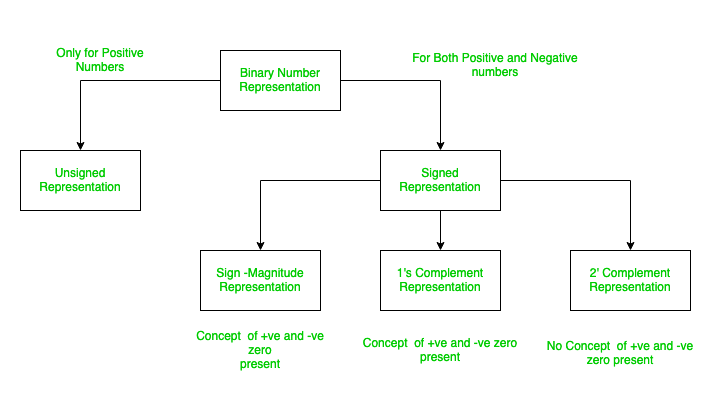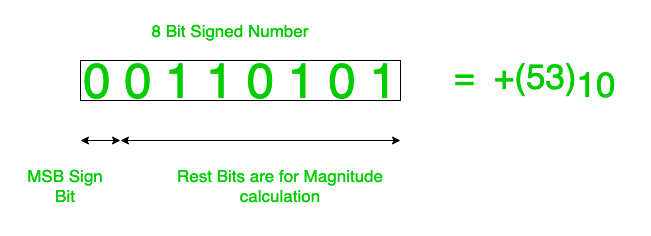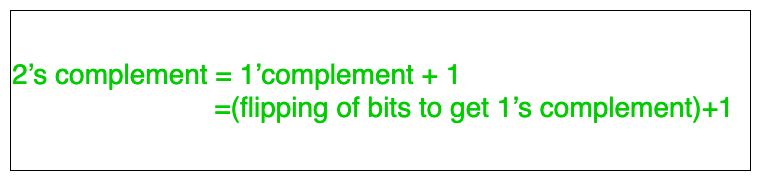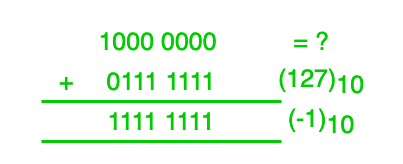# Basics of Signed Binary numbers of ranges of different Datatypes

Core concepts of Signed Binary Numbers  And Explanation of Ranges of Different Datatypes. If I would ask what is the value of (1000 0000)2 in decimal then certainly two ambiguous answers will come up (-128)10 and (+128)10 .

So the concept of signed and unsigned numbers comes up to help overcome the ambiguity.

Now if it is given to be unsigned number the  (+128)10 is the correct answer because there is no sign bit in case of unsigned numbers. Thus, here the MSB(Most Significant Bit ) is not reserved to represent sign of number.

But in case it is given that it is signed number, the  (-128)10  is the correct answer.
In case of signed numbers the MSB is reserved to represent the sign of the number.
Thus if the number is of n bits, then in this 1 bit is used for representing sign of the number and rest (n-1) bits are used to represent the magnitude of the number.

Methods to represent a Signed number:

• Sign Magnitude Form
• 1’s Complement Form
• 2’s Complement FormThese are explained as following below.

1. Sign Magnitude Form:
Here, the MSB is reserved for signed bit, by using rest (n-1)bits we can directly get the decimal value using the normal formula of binary to decimal conversion(by multiplying 2i where i represents index position from LSB(Least Significant Bit)). Note that the index starts from 0 not 1 from LSB side.

2. 1’s complement Form:
Here, the MSB is reserved for signed bit, and rest (n-1)bits are stored in form 1’s complement of the number.What I mean to say will be clear from the below example:

Consider to represent -7 using 4 bits in 1’s complement.
Here given, 4 bits so MSB 1 bit reserved to represent sign. So, now we are left with 3 bits.
And we know (+7)10=(1112.

But it is given to store the number in 1’s complement form thus, 1’s complement of (+7) is calculated.
1’s complement of (+7)10=(000)2. (As 1’s complement is calculated for flipping the zeros to ones and vice-versa).

Thus, finally 1’s complement representation of (-7)10=(1000)2. (using 4 bits).

3. 2’s complement Form:
Here, the MSB is reserved for signed bit, and rest (n-1) bits are stored in form 2’s complement of the number.

What I mean to say will be clear from the below example:

Consider the same example explained above i.e., represent -7 using 4 bits but now in 2’s complement.
Here given, 4 bits so MSB 1 bit reserved to represent sign.So, now we are left with 3 bits.
And we know (+7)10=(111)2.

But it is given to store the number in 2’s complement form thus, 2’s complement of (+7) is calculated.
1’s complement of (+7)10=(001)2.(As 2’s complement is calculated for flipping the zeros to ones and vice-versa) and adding 1 to the result we get after flipping the bits.

In other words,Thus, finally 2’s complement representation of (-7)10=(1001)2.(using 4 bits).

But wait there is ambiguity, how can (-128)10=(1000 0000)2 in 8 bit 2’s form signed number?

But the trick is here, let us think that we don’t know what (1000 0000)2 represents and add (+127)10 to it and after adding the result we got is (1111 1111)2= (-1)10. Thus, the only possible value of (1000 0000)2  is (-128)10 in 8 bit 2’s form signed number.

Now, this concept is allowed in 2’s complement because in 2’s complement there is concept of (negative 0). If we want to calculate by taking 2’s complement of (1000 0000)2 then after ignoring the MSBs(sign bit) the 1’s complement of number is (111 1111) and 2’s complement is(000 0000). Thus magnitude is 0 and sign is negative .Decimal number we should get is (-0) but as I have already said there is no concept of (negative 0) in 2’s complement.

Thus, this confirms (-128)10=(1000 0000)2.Ranges in data types in programming Languages can be explained with this. Now we all that short (data type) in c++/java is of 2 bytes i.e 16  bits and we say range is from ( -32, 768 to 32, 767 ). Now, just notice that positive number range is till -32, 768  but positive number is only till 32, 767 i.e negative range is more than positive number range(talking about the magnitude of both sides only) but you might be thinking that both the positive and negative ranges should be same but here they are not same.

Confused why so?
The reason for the same is the concept as I explained above thus negative range is one more than positive range (1000 0000 0000 0000)2 is (-32, 768)10. This concept can be extended to all data types and is the reason behind the ranges of all data types we use in our programming languages.

Attention reader! Don’t stop learning now. Get hold of all the important CS Theory concepts for SDE interviews with the CS Theory Course at a student-friendly price and become industry ready.

My Personal Notes arrow_drop_upCheck out this Author's contributed articles.

If you like GeeksforGeeks and would like to contribute, you can also write an article using contribute.geeksforgeeks.org or mail your article to contribute@geeksforgeeks.org. See your article appearing on the GeeksforGeeks main page and help other Geeks.

Please Improve this article if you find anything incorrect by clicking on the "Improve Article" button below.